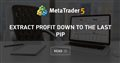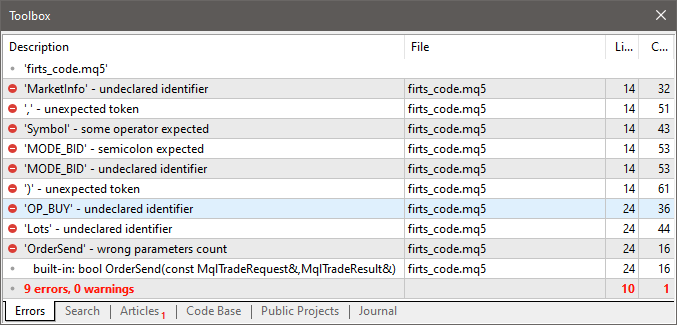# Unexpected end of program and unbalanced parentheses in my code

Hey I wrote this code that I thought it would be simple but I'm having this two errors (Unexpected end of program and unbalanced parentheses) and I don't know how to solve it. This is my first code  so if someone could help me I would be so grateful. It's a MQL5 code.

Thanks :)

```// This EA will open a buy order when the price of the asset falls at least 5 points, take profits when there are 4 points of profit and set a stop loss at 5 points of loss.

//inputs:
extern double takeProfit = 4.0;
extern double stopLoss = 5.0;

//variables
double openPrice;

// OnTick function
void OnTick()
{
// Get the current price
double currentPrice = MarketInfo(Symbol(), MODE_BID);

// Check if the open price has been set
if (openPrice == 0)
{
// Check if the price has fallen at least 5 points
if (currentPrice < (openPrice - 5.0))
{
openPrice = currentPrice;
OrderSend(Symbol(), OP_BUY, Lots, currentPrice, 0, 0, 0, "My EA", 0, 0, Green);
}
}

// Check if there is an open position
if (PositionSelect(Symbol()))

```Extract profit down to the last pip
• www.mql5.com
The article describes an attempt to combine theory with practice in the algorithmic trading field. Most of discussions concerning the creation of Trading Systems is connected with the use of historic bars and various indicators applied thereon. This is the most well covered field and thus we will not consider it. Bars represent a very artificial entity; therefore we will work with something closer to proto-data, namely the price ticks.

Please EDIT your post (don't create a new one) and post your code properly (and eliminate any unnecessary empty lines) ...

Joaquín Defranza: Hey I wrote this code that I thought it would be simple but I'm having this two errors (Unexpected end of program and unbalanced parentheses) and I don't know how to solve it. This is my first code  so if someone could help me I would be so grateful. It's a MQL5 code. I let the code here and in files. Thanks :)

Here are some tips ...

```// This EA will open a buy order when the price of the asset falls at least 5 points,
// take profits when there are 4 points of profit and set a stop loss at 5 points of loss.

//inputs:
extern double takeProfit = 4.0;
extern double stopLoss = 5.0;

//variables
double openPrice; // <- Uninitialised global variable

// OnTick function
void OnTick()
{
// Get the current price
double currentPrice = MarketInfo(Symbol(), MODE_BID); // <- This is MQL4 code, not MQL5 code

// Check if the open price has been set
if (openPrice == 0) // <- using a variable before it is initialised
{
// Check if the price has fallen at least 5 points
if (currentPrice < (openPrice - 5.0))
{
openPrice = currentPrice;
OrderSend(Symbol(), OP_BUY, Lots, currentPrice, 0, 0, 0, "My EA", 0, 0, Green); // <- This is MQL4 code, not MQL5 code
}
}

// Check if there is an open position
if (PositionSelect(Symbol()))
{ // <- You were missing the code block braces
// <- Where is the rest of the code?
} // <- You were missing the code block braces
} // <- You were missing the closing brace for the OnTick()```

However, there are still errors after correcting the braces, mainly because of the unrecognised MQL4 code ...That's MQL4 not MQL5, in MQL5 there is no MarketInfo function, OP_BUY, and OrderSend doesn't work in the same way
Reason: